## Explorative data analysis with pandas¶

### Pandas Series¶

• Series is a one-dimensional labeled array capable of holding any data type (integers, strings, floating point numbers, Python objects, etc.).
• Series is ndarray-like; Series is dict-like
• The axis labels are collectively referred to as the index.

http://pandas.pydata.org/pandas-docs/stable/dsintro.html

In :
import pandas as pd
data = [2,4,3,4,4]
index = range(5)
s = pd.Series(data)#, index=index)
s

Out:
0    2
1    4
2    3
3    4
4    4
dtype: int64

### Pandas Data Frames¶

DataFrame is a 2-dimensional labeled data structure with columns of potentially different types.

In :
import matplotlib
import matplotlib.pyplot as plt
import numpy as np
%matplotlib inline
matplotlib.style.use('ggplot')

In :
# from http://www.analyticsvidhya.com/blog/2014/08/baby-steps-python-performing-exploratory-analysis-python/

# load the data into a python data frame
#type(df)

Out:
PassengerId Survived Pclass Name Sex Age SibSp Parch Ticket Fare Cabin Embarked
0 1 0 3 Braund, Mr. Owen Harris male 22 1 0 A/5 21171 7.2500 NaN S
1 2 1 1 Cumings, Mrs. John Bradley (Florence Briggs Th... female 38 1 0 PC 17599 71.2833 C85 C
In :
#generate various summary statistics
df.describe()

Out:
PassengerId Survived Pclass Age SibSp Parch Fare
count 891.000000 891.000000 891.000000 714.000000 891.000000 891.000000 891.000000
mean 446.000000 0.383838 2.308642 29.699118 0.523008 0.381594 32.204208
std 257.353842 0.486592 0.836071 14.526497 1.102743 0.806057 49.693429
min 1.000000 0.000000 1.000000 0.420000 0.000000 0.000000 0.000000
25% 223.500000 0.000000 2.000000 20.125000 0.000000 0.000000 7.910400
50% 446.000000 0.000000 3.000000 28.000000 0.000000 0.000000 14.454200
75% 668.500000 1.000000 3.000000 38.000000 1.000000 0.000000 31.000000
max 891.000000 1.000000 3.000000 80.000000 8.000000 6.000000 512.329200
In :
df.Age.hist(bins=20)

Out:
<matplotlib.axes._subplots.AxesSubplot at 0x10e21f650>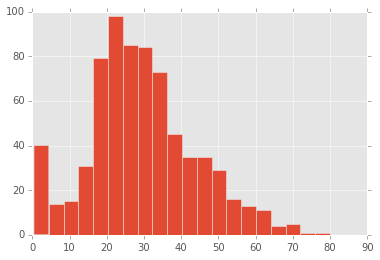Age has (891–714=) 277 missing values.

We can also look that about 38% passangers survived the tragedy. How? The mean of survival field is 0.38 (Remember, survival has value 1 for those who survived and 0 otherwise)

By looking at percentiles of Pclass, you can see that more than 50% of passengers belong to class 3,

The age distribution seems to be in line with expectation. Same with SibSp and Parch

The fare seems to have values with 0 indicating possibility of some free tickets or data errors. On the other extreme, 512 looks like a possible outlier / error

### Level of measurements¶

• Nominal
• Ordinal
• Interval
• Ratio

#### Data distributions:¶

There are 3 variety of measures, required to understand a distribution:

• Measure of Central tendency
• Measure of dispersion
• Measure to describe shape of curve

#### Measures of Central tendency¶

In :
#    Mean – or the average
print "Mean of Age:", df['Age'].mean()

#    Median – the value, which divides the population in two half
print "Media of Age:", df['Age'].median()

#    Mode – the most frequent value in a population
print "Mode of Pclass:", df['Pclass'].mode()

# df.Pclass.min()

Mean of Age: 29.6991176471
Media of Age: 28.0
Mode of Pclass: 0    3
dtype: int64
1


#### Measure of dispersion¶

• Range: Difference in the maximum and minimum value in the population
• Quartiles: Values, which divide the populaiton in 4 equal subsets (typically referred to as first quartile, second quartile and third quartile)
• Inter-quartile range – The difference in third quartile (Q3) and first quartile (Q1). By definition of quartiles, 50% of the population lies in the inter-quartile range.
• Variance: The average of the squared differences from the Mean.
• Standard Deviation: is square root of Variance

#### Measures to describe shape of distribution:¶

• Skewness: Skewness is a measure of the asymmetry. Negatively skewed curve has a long left tail and vice versa.
• Kurtosis: Kurtosis is a measure of the “peaked ness”. Distributions with higher peaks have positive kurtosis and vice-versa
In :
print "Skew of age: ", df.Age.skew()
print "Kurtosis of age:", df.Age.kurtosis()

Skew of age:  0.389107782301
Kurtosis of age: 0.178274153642

In :
#Returns first n rows

Out:
PassengerId Survived Pclass Name Sex Age SibSp Parch Ticket Fare Cabin Embarked
0 1 0 3 Braund, Mr. Owen Harris male 22 1 0 A/5 21171 7.2500 NaN S
1 2 1 1 Cumings, Mrs. John Bradley (Florence Briggs Th... female 38 1 0 PC 17599 71.2833 C85 C
2 3 1 3 Heikkinen, Miss. Laina female 26 0 0 STON/O2. 3101282 7.9250 NaN S
In :
# not all summary statistics is displayed by describe(), e.g. the median
df['Age'].median()

Out:
28.0
In :
df['Sex'].unique()

Out:
array(['male', 'female'], dtype=object)
In :
# Histogram with matplotlib
fig = plt.figure(figsize=(6,6))
ax.hist(df['Age'], bins = 15, range = (df['Age'].min(),df['Age'].max()))
# or method hist() of a pandas series
# df['Age'].hist(bins = 10)
plt.title('Age distribution')
plt.xlabel('Age')
plt.ylabel('Count of P assengers')
plt.show()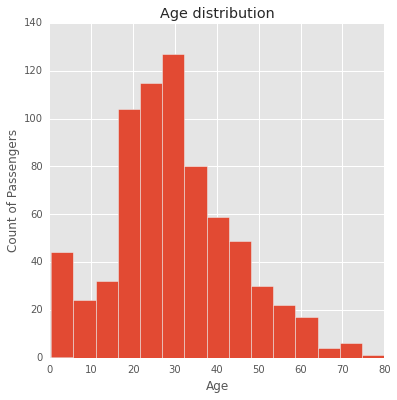In :
##
fig = plt.figure()
ax.hist(df['Fare'], bins = 10, range = (df['Fare'].min(),
50.))
#df['Fare'].max()))
plt.title('Fare distribution')
plt.xlabel('Fare')
plt.ylabel('Count of Passengers')
plt.show()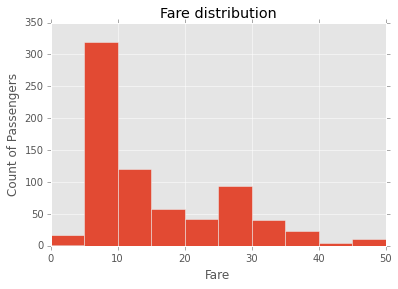In :
df.boxplot(column='Fare', by = 'Pclass')

Out:
<matplotlib.axes._subplots.AxesSubplot at 0x109d19d10>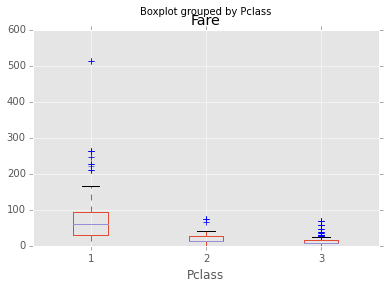In :
import seaborn as sns
sns.jointplot("Age", "Fare", df, kind='reg')

Out:
<seaborn.axisgrid.JointGrid at 0x10c60cb90>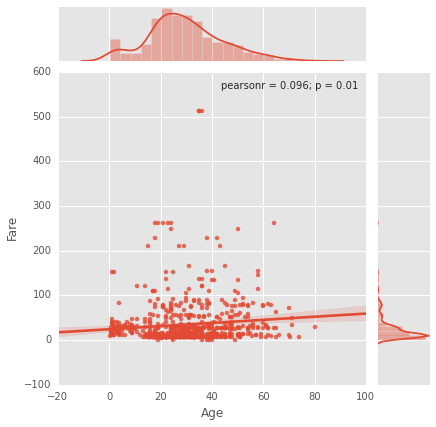In :
sns.lmplot("Age", "Fare", df, col="Pclass")

Out:
<seaborn.axisgrid.FacetGrid at 0x10d7e8b90>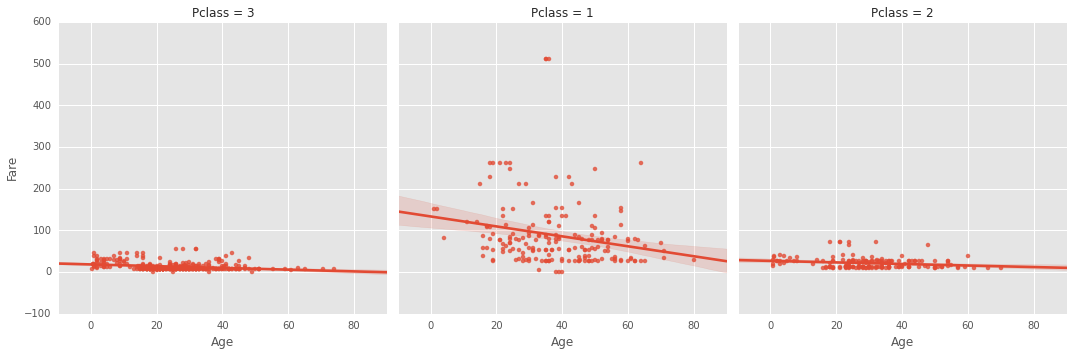In :
## Violine Plot

df_nn = df[pd.notnull(df['Age'])]
import seaborn as sns
sns.violinplot(df_nn['Age'], df_nn['Sex'], cut=0.) #Variable Plot
sns.despine()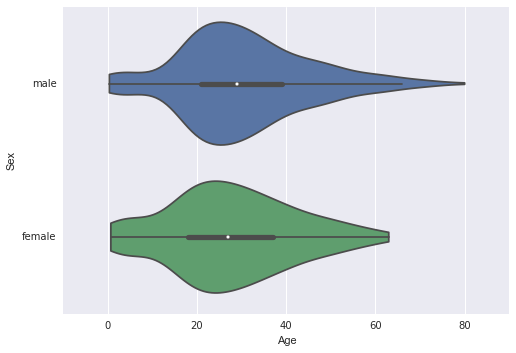In :
fig = plt.figure(figsize=(8,4))
ax.axis("equal")
plt.title("Pclass distribution")
pgroup = df.groupby(['Pclass'])
pcouts = pgroup.PassengerId.count()
pcouts.name = "Number of Passengers per Class"
pcouts.plot(kind='Pie', autopct="%1.1f%%", ax=ax)

Out:
<matplotlib.axes._subplots.AxesSubplot at 0x109d40e50>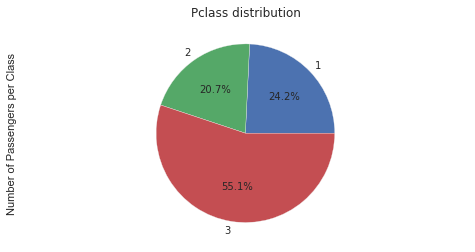In :
temp1 = df.groupby('Pclass').Survived.count()
# or temp1 = df['Survived'].groupby(df['Pclass']).count()

temp2 = df.groupby('Pclass').Survived.sum()/df.groupby('Pclass').Survived.count()
fig = plt.figure(figsize=(8,4))
ax1.set_xlabel('Pclass')
ax1.set_ylabel('Count of Passengers')
ax1.set_title("Passengers by Pclass")
temp1.plot(kind='bar')

temp2.plot(kind = 'bar')
ax2.set_xlabel('Pclass')
ax2.set_ylabel('Probability of Survival')
ax2.set_title("Probability of survival by class")

Out:
<matplotlib.text.Text at 0x10b96ec10>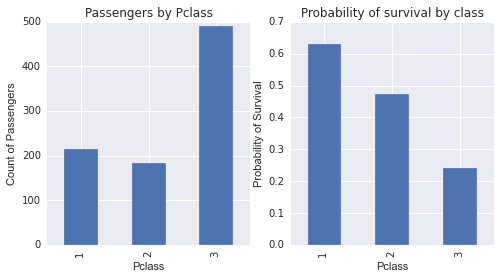In :
# binning can be done with pandas directly
# Categorical
age_bins = pd.cut(df.Age, 8, precision=0)
groups = df.groupby(age_bins)
groups.PassengerId.count().plot(kind='bar')
temp = groups.Survived.mean()
temp.plot(kind='bar')
group_names = ['kids', 'youth', 'adults', 'seniors']
age_bins = pd.cut(df.Age, [df.Age.min(), 14, 22, 55, df.Age.max()], labels = group_names)
groups = df.groupby(age_bins)
temp = groups.Survived.mean()
temp.plot(kind='bar')

Out:
<matplotlib.axes._subplots.AxesSubplot at 0x10b93b750>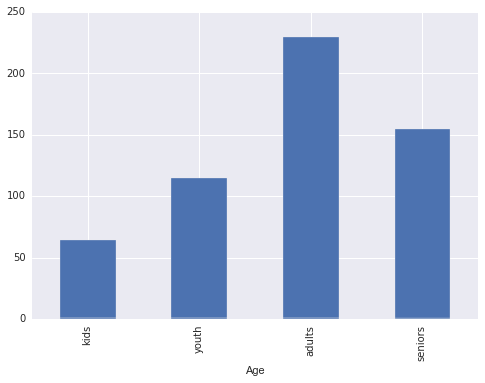In :
# for discretize variable into equal-sized buckets based on rank or based on sample quantiles: see qcut
#pd.qcut?

In :
## binning with numpy
#bins = np.round(np.linspace(0., df.Age.max(), 10))
#age_bins = pd.Series(np.digitize(df.Age, bins))
##the last bin is here nan
#age_bins[age_bins == age_bins.max()] = 'NaN'
#for i in range(len(bins)-1):
#  age_bins[age_bins == i+1] = "{0:2.0f}-{1:2.0f}".format(bins[i], bins[i+1])
#groups = df.groupby(age_bins)
#temp = groups.Survived.mean()
#temp.plot(kind='bar')

In :
data = df.groupby([age_bins,'Sex']).Survived.mean()
# Note: data has a hierachical index
data

fig = plt.figure(figsize=(8,4))
ax.set_xlabel('Age')
ax.set_ylabel('Probability of Survival')
ax.set_title("Probability of survival by age and sex")
data.unstack(level=1).plot(kind='bar', subplots=False, ax=ax)

Out:
<matplotlib.axes._subplots.AxesSubplot at 0x10d3f60d0>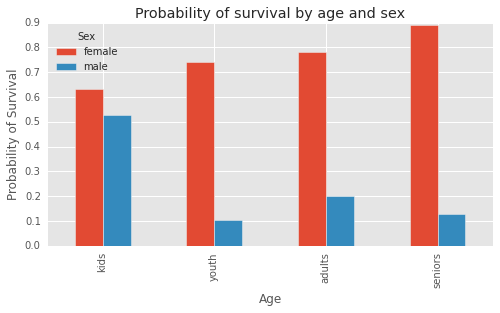In :
var = df.groupby(['Sex','Survived']).PassengerId.count()
ax = var.unstack().plot(kind='bar',stacked=True,  color=['red','blue'], grid=False)
ax.set_xlabel('Sex')
ax.set_ylabel('Number of Passengers')

Out:
<matplotlib.text.Text at 0x10ec01d90>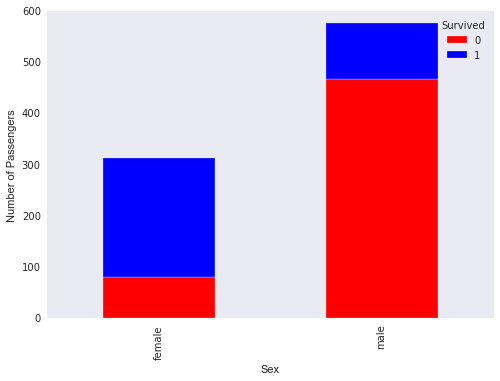In :
from statsmodels.graphics.mosaicplot import mosaic

_ = mosaic(df, ['Survived', 'Sex', 'Pclass'])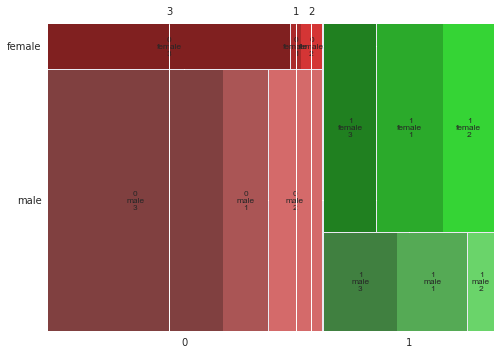In :
df['Adult'] = df["Age"].apply(lambda age: "adult" if age >14. else "child")
_ = mosaic(df, ['Survived', 'Sex', 'Pclass', 'Adult'])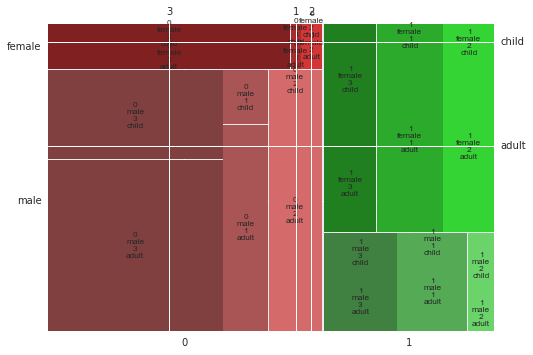In :
#) Probability of surviving of a woman in the 3. class?
df[(df['Sex']=='female') & (df['Pclass']==3)].Survived.mean()

Out:
0.5
In :
# more plots with pandas see http://pandas.pydata.org/pandas-docs/stable/visualization.html

In :
# Some simple string handling for getting the salutations
salutation = df.Name.apply(lambda w: w.split(',').split('.').strip())

# df.Saluation = ... Doesn't work here properly, because the column doesn't exists right now !!
df['Salutation'] = salutation
df.groupby('Salutation').PassengerId.count()
# use 'Other' for the low count salutations
df.Salutation [np.invert( (df.Salutation == 'Mr') | (df.Salutation == 'Mrs') | (df.Salutation == 'Miss') | (df.Salutation == 'Master') )]  = 'Other'
df.boxplot(column='Age', by = 'Salutation')

/Users/christian/.virtualenvs/standard/lib/python2.7/site-packages/ipykernel/__main__.py:10: SettingWithCopyWarning:
A value is trying to be set on a copy of a slice from a DataFrame

See the caveats in the documentation: http://pandas.pydata.org/pandas-docs/stable/indexing.html#indexing-view-versus-copy

Out:
<matplotlib.axes._subplots.AxesSubplot at 0x109d4fa10>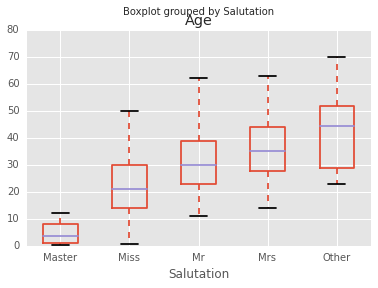In :
################
# Fill missing values with pandas (alternative: scikit learn imputter)
# After the explorative data analysis!

medianAge = df.Age.median()
df.Age = df.Age.fillna(medianAge)
##################